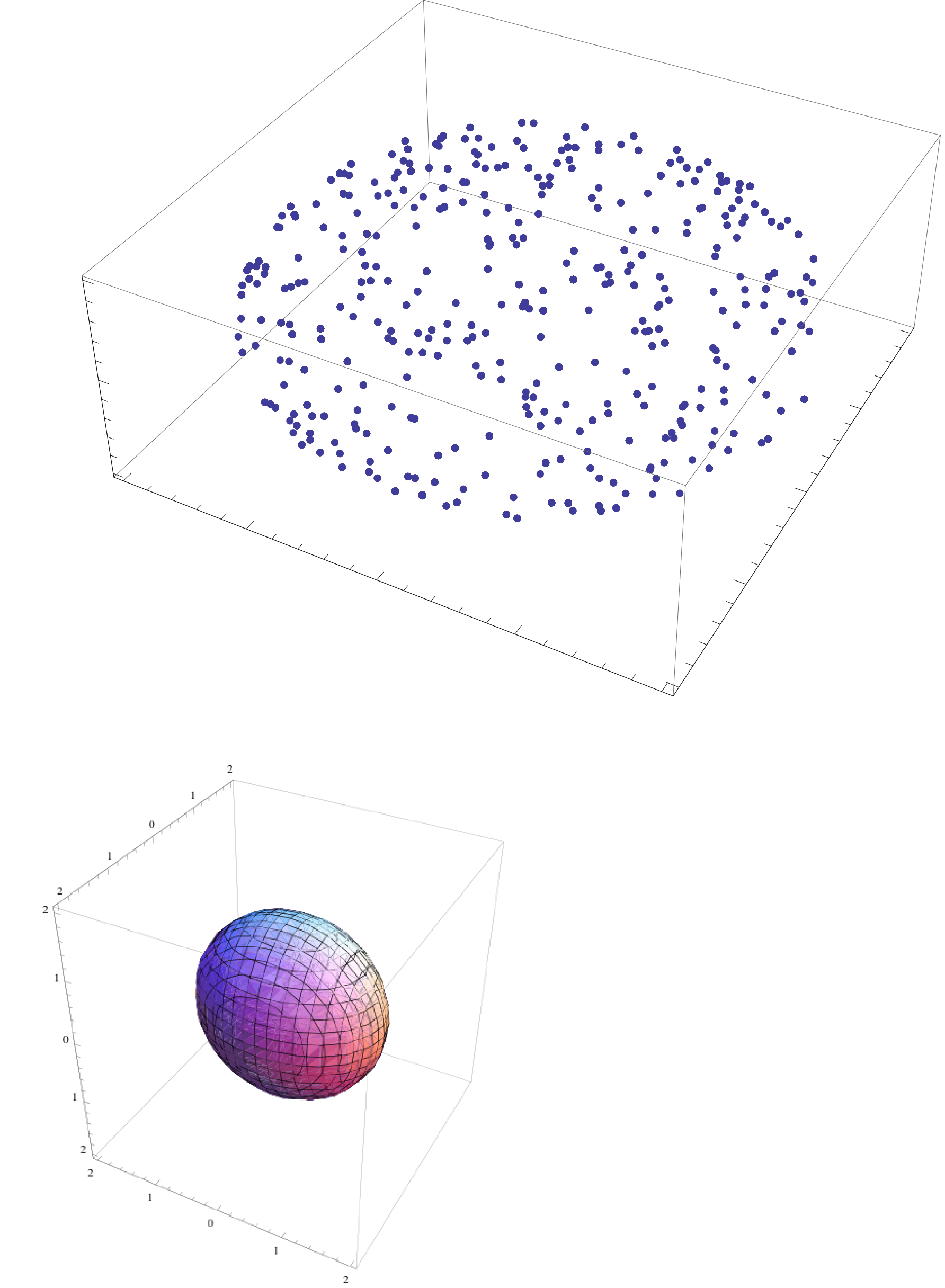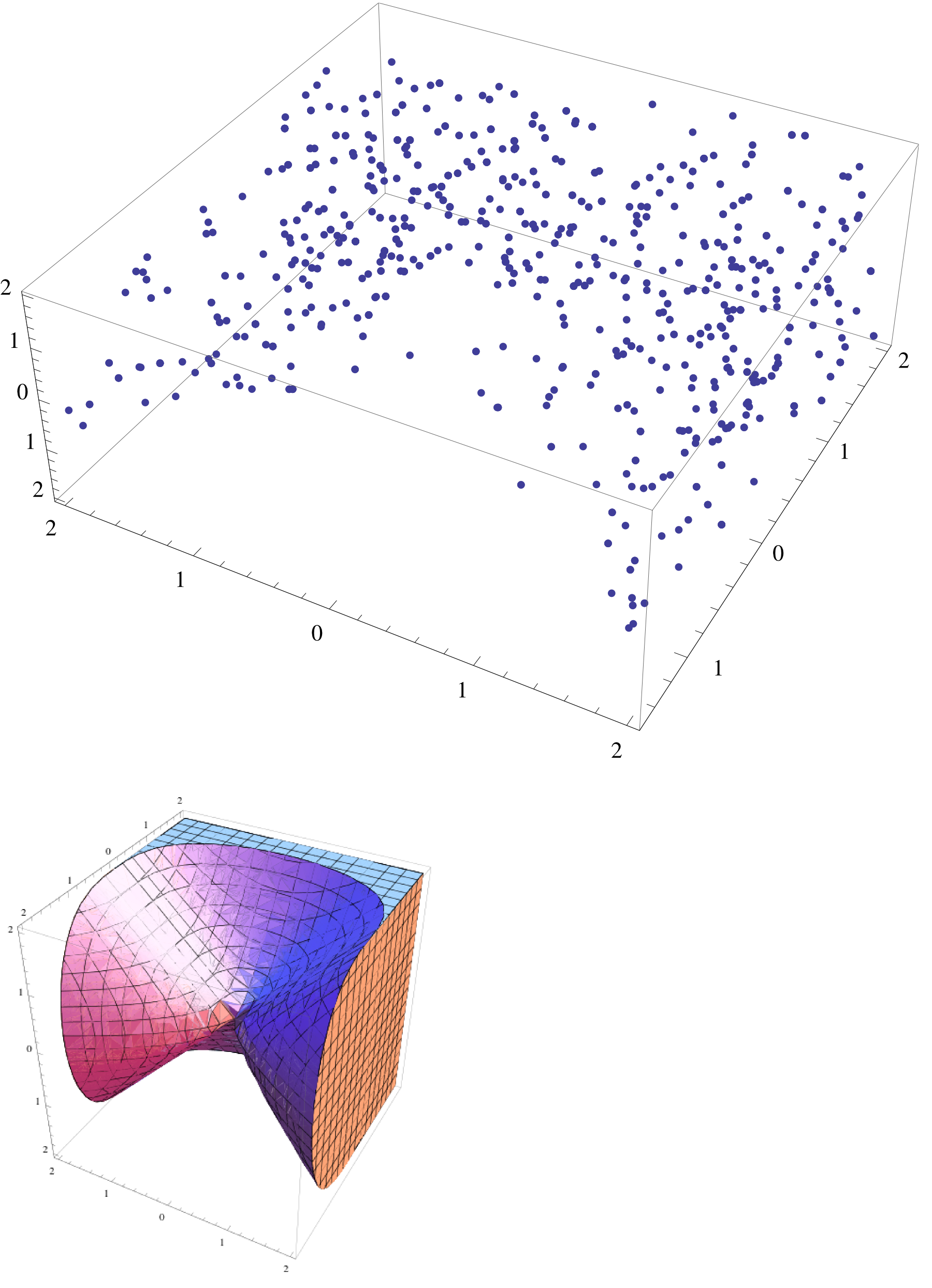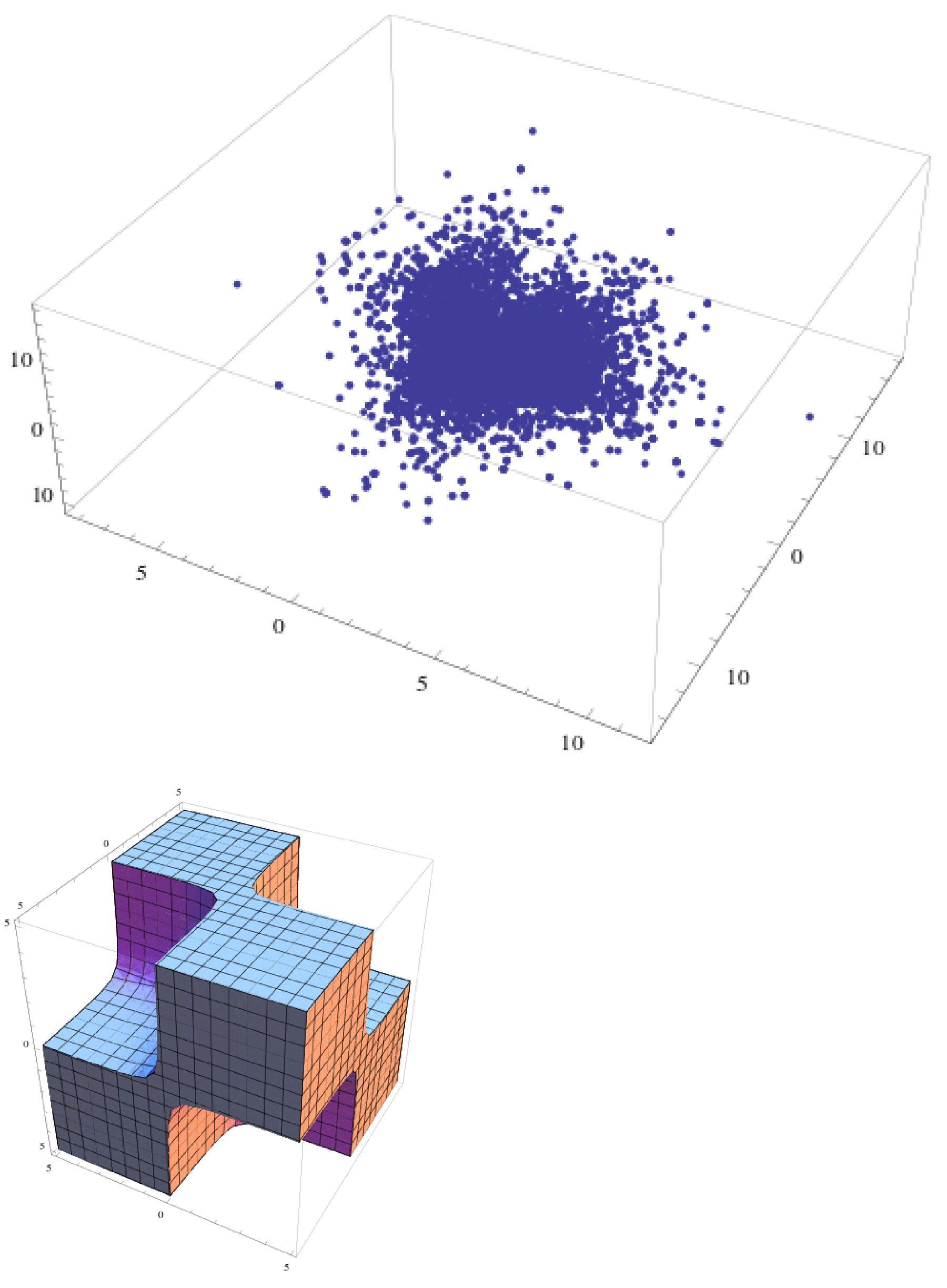Zenna Tavares

Computer Assisted Programming Group (PI: Armando Solar Lezama)
Department of Brain and Cognitive Sciences
CSAIL

JuliaCon 6/25/2015

## What is Probabilistic Programming?

J Jara-Ettinger, L Schulz, JB Tenenbaum (2015).## Example: Inverse Planning## Parameter Estimation

### Infer rate parameter of exponential distribution$$\begin{split} \lambda &\sim \mathrm{Uniform}(0, 2)\\ x_n &\sim \mathrm{Exponential}(λ)\\ observe \; \mathbf{x} &= [0.083, 0.55, 2.37]\\ \end{split}$$

## Conditional Samples

priorposterior## Inference Queries

### Conditional sampling with rand

λ = uniform(0,2)
x1 = exponential(λ)
x2 = exponential(λ)
x3 = exponential(λ)
observations = (x1 == 0.083) & (x2 == 0.55) & (x3 == 2.37)

rand(λ, observations, 100)


## Conditional Sampling is Not Easy

### probability of observation == true becomes vanishingly small

dreamreality## Random Variables are not Random!

### They are functions

λ = uniform(0,2)
typeof(λ) => RandVar{Float64}

function λ(ω) = ω * 2 end  # input ω ∈ [0, 1]

λ(0.1) => 0.2  # x is just a function

λ = randunif()
λ(ω) => 0.6923295214 # to sample: call with uniformly sampled input


## Pointwise Random Variable Algebra

$$\begin{split} Z &= X + Y\\ Z(\omega) &= X(\omega) + Y(\omega) \end{split}$$## Pointwise Random Variable Algebra

a = exponential(0.5); b = exponential(0.5)
c = a + b
typeof(c) => RandVar{Float64}
rand(c, 5) => [3.425, 2.990, 9.670, 0.45759, 3.6357]

d = c + 100
typeof(d) => RandVar{Float64}
rand(d, 5) => [104.883, 103.50, 100.50, 100.485, 100.549]

e = exponential(d)
typeof(e) => RandVar{Float64}
rand(e, 5) => [0.020, 0.0043, 0.00108, 0.0041, 0.0052]


## Single Sample Space

### Random Variables are dependent through $$\Omega$$ (Euclidean unit cube)## Single Sample Space

### Random Variables are dependent through $$\Omega$$ (Euclidean unit cube)## Single Sample Space

### Random Variables are dependent through $$\Omega$$ (Euclidean unit cube)## Complete Example using Random Arrays

### are arrays of RandVars

rand(X::RandVar{T}, Y::RandVar{Bool}, n::Integer) = ...

type RandArray{T,N} <: DenseArray{RandVar{T},N} ...

λ = uniform(0,2)
x = mvexponential(λ, 3)
observations = x == [0.083, 0.55, 2.37]

rand(x, observations, 100)


## Single Sample Space

### Random Variables are dependent through $$\Omega$$ (Euclidean unit cube)## Conditional Sampling is Easy

### Guess (probabilistically) and check until condition is satisfied

$$P(A|B) = \frac{P(A \cap B)}{P(B)}$$
function rand(X::RandVar, Y::RandVar{Bool})
while true
ω = rand_unif_vector()
if Y(ω)
return X(ω)
end
end
end


## Conditional Sampling is Easy

### Guess (probabilistically) and check until condition is satisfied

$$P(A|B) = \frac{P(A \cap B)}{P(B)}$$
function rand(X::RandVar, Y::RandVar{Bool})
while true
ω = rand_unif_vector()
if Y(ω)
return X(ω)
end
end
end


## Conditional Sampling is Not Easy

### probability Y(ω) == true becomes vanishingly small

dreamreality## Consider sets instead of single points

### and let an Oracle (constraint solver) do the hard work$$\mathcal{O}_Y(A) = \left\{ \begin{array}{lr} T & : A \subseteq Y\\ F & : A \cap Y = \varnothing\\ TF & : Otherwise\\ \end{array} \right.$$

## Play a game with the Oracle

### Split and randomly choose one side## δ-Relaxation

### Make thin lines thick - (S Gao, J Avidad, EM Clarke - 2012)

$$Y=X$$$$Y-X<\delta$$## Example: Geometric Sampling

### Sample from surface/interior of geometric objects## Sigma and Julia

The Good

• simplicity: model-algorithm separated
• type hierarchy: use RandVars in existing code
• runtime compilation

The Ugly

• types: RandVar{Float64} <: FloatingPoint ?
• control flow breaks things: if, while, for, &, |, ...

## Conclusion

What is Probabilistic Programming?

• Bayesian and Conditional Inference are same-same but different
• Sigma is requires complete model - no black boxes
• One algorithm to rule them all?

Do we need new languages?

• Not really, no
• Maybe... Uncertain type Inference? Probabilistic parsing?

## Thank You!

Contributors Welcome! - https://github.com/zenna/Sigma.jl